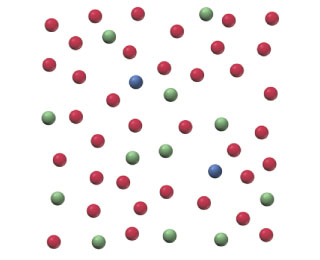# Problem: Below is a representation of 50 atoms of a fictitious element called westmontium (Wt). The red spheres represent Wt-296, the blue spheres Wt-297, and the green spheres Wt-298. The mass of each Wt isotope is measured relative to C-12 and tabulated below. Use the mass of C-12 to convert each of the masses to amu and calculate the atomic mass of Wt.IsotopeMassWt-29624.6631 12 C)Wt-29724.7486 12 C)Wt-29824.8316 12 C)

###### FREE Expert Solution

Mass Wt-296 = 24.6631 x 12 amu = 295.9572 amu

Mass Wt-297 = 24.7486 x 12 amu = 296.9832 amu

Mass Wt-298 = 24.8316 x 12 amu = 297.9792 amu

79% (25 ratings)###### Problem Details

Below is a representation of 50 atoms of a fictitious element called westmontium (Wt). The red spheres represent Wt-296, the blue spheres Wt-297, and the green spheres Wt-298.The mass of each Wt isotope is measured relative to C-12 and tabulated below. Use the mass of C-12 to convert each of the masses to amu and calculate the atomic mass of Wt.

 Isotope Mass Wt-296 24.6631 12 C) Wt-297 24.7486 12 C) Wt-298 24.8316 12 C)

Frequently Asked Questions

What scientific concept do you need to know in order to solve this problem?

Our tutors have indicated that to solve this problem you will need to apply the Atomic Mass concept. You can view video lessons to learn Atomic Mass. Or if you need more Atomic Mass practice, you can also practice Atomic Mass practice problems.

What professor is this problem relevant for?

Based on our data, we think this problem is relevant for Professor Michael's class at UNC.